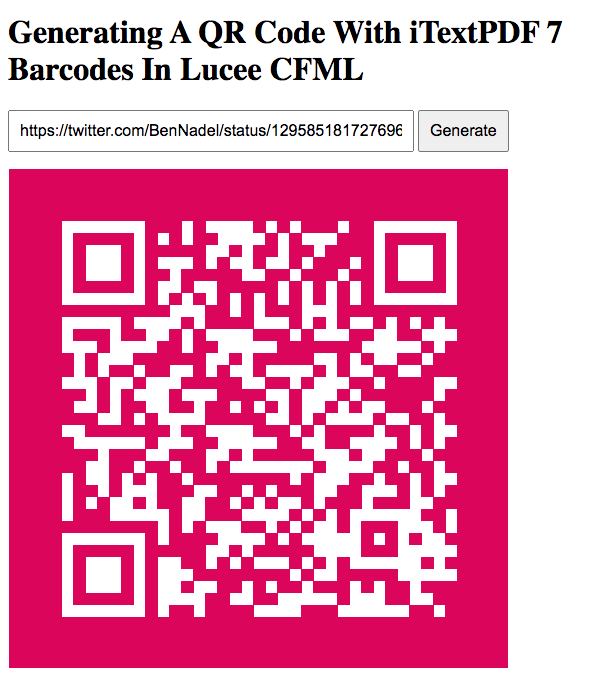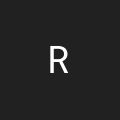# Generating A QR Code With iTextPDF 7 Barcodes In Lucee CFML 5.3.6.61

By on
Tags:

At InVision, my teammate Josh Siok has been experimenting with the use of QR Codes as a means to send prototypes to a user's mobile device. I know of QR Codes; but, I've never generated one before. As such, I wanted to see if I could generate a QR Code in ColdFusion. To this end, I came across Tim Cunningham's QRToad library which uses iTextPDF 5 under the hood. However, the latest version of iTextPDF is 7.1.13, which has a different API. As, I wanted to see if I could generate a QR Code with iTextPDF 7 in Lucee CFML 5.3.6.61.

Tim's QRToad library works by generating an iTextPDF QR Code raster image which he then draws to a ColdFusion Image object through the underlying Java API. One major difference that I can see between the v5 and v7 iTextPDF API is that I can no longer provide a width and height to the `BarcodeQRCode` class. As such, when I go to generate a QR Code using the same strategy, the resultant QR Code image was tiny - something like 30-pixels across.

After digging around in the Java Docs a bit more, I discovered the `QRCodeWriter` class, which does take dimensions. However, instead of returning an Image, the `QRCodeWriter` class returns a `ByteMatrix`, which is two-dimensional array of On/Off pixel flags. Of course, in ColdFusion, we have the ability to draw points on an Image object canvas; so, I decided to try drawing the pixel values as individual points on a ColdFusion Image:

``````<cfscript>

qrCodeImage = generateQrCode( url.value, 500, 500, "e0005a" )
.write( "./qr-code.png", 1, true )
;

// ------------------------------------------------------------------------------- //
// ------------------------------------------------------------------------------- //

/**
* I generate a QR Code that encodes the given value. The width and height represent
* the minimum dimensions of the rendered image; but, the QR Code may exceed the given
* dimensions if the minimum does not provide enough space to encode the given value.
*
* @value I am the URL to encode in the QR Code.
* @minWidth I am the minimum width of the QR Code image.
* @minHeight I am the minimum height of the QR Code image.
*/
public struct function generateQrCode(
required string value,
required numeric minWidth,
required numeric minHeight,
required string color,
string backgroundColor
) {

var byteMatrix = getByteMatrix( value, minWidth, minHeight );

// Note that we are NOT using the minWidth / minHeight values when calculating
// the QR Code image dimensions. That's because those values were MINIMUM values
// that may have been exceeded. As such, we have to get the dimensions of the
// QR Code image from the generated matrix.
var qrCodeWidth = byteMatrix.getWidth();
var qrCodeHeight = byteMatrix.getHeight();
var qrCodeImage = imageNew( "", qrCodeWidth, qrCodeHeight, "argb" )
.setAntialiasing( "off" )
;

// If a background color was provided, paint over the entire canvas.
if ( ! isNull( backgroundColor ) ) {

qrCodeImage
.setDrawingColor( backgroundColor )
.drawRect( 1, 1, qrCodeWidth, qrCodeHeight, true )
;

}

qrCodeImage.setDrawingColor( color );

// The ByteMatrix is a two-dimensional array of ON/OFF pixel values. Let's
// iterate over the ByteMatrix and draw a point for every ON pixel.
loop
index = "local.y"
item = "local.row"
array = byteMatrix.getArray()
{

loop
index = "local.x"
item = "local.pixelValue"
array = row
{

if ( pixelValue ) {

qrCodeImage.drawPoint( x, y );

}

}

}

return( qrCodeImage );

}

/**
* I generate a ByteMatrix (two-dimensional array of pixel-data) for a QR Code that
* encodes the given value. The min width and height set the size of the QR Code; but,
* the size may exceed the given dimensions if more room is needed to encode the
* value. With the ByteMatrix, a zero is "no color" and a non-zero (-1 or 1) is a
* colored-in location.
*
* @value I am the URL to encode in the QR Code.
* @minWidth I am the minimum width of the QR Code image.
* @minHeight I am the minimum height of the QR Code image.
*/
public any function getByteMatrix(
required string value,
required numeric minWidth,
required numeric minHeight
) {

var jarFiles = [
expandPath( "./barcodes-7.1.13.jar" )
// CAUTION: Maven said that the following JAR files were compile dependencies
// of the iText library. However, it seems that I can generate the ByteMatrix
// for the QR Code without including them.
// --
// expandPath( "./bcpkix-jdk15on-1.64.jar" ),
// expandPath( "./bcprov-jdk15on-1.64.jar" ),
// expandPath( "./io-7.1.13.jar" ),
// expandPath( "./kernel-7.1.13.jar" )
];

var writer = createObject( "java", "com.itextpdf.barcodes.qrcode.QRCodeWriter", jarFiles );

return( writer.encode( value, minWidth, minHeight ) );

}

</cfscript>
<cfoutput>

<!doctype html>
<html lang="en">
<meta charset="utf-8" />
<body>

<h1>
Generating A QR Code With iTextPDF 7 Barcodes In Lucee CFML
</h1>

<form method="get">
<input
type="text"
name="value"
value="#encodeForHtmlAttribute( url.value )#"
size="47"
style="font-size: inherit ; padding: 10px ;"
/>
<button type="submit" style="font-size: inherit ; padding: 10px ;">
Generate
</button>
</form>

<p>
<img src="./qr-code.png" />
</p>

</body>
</html>

</cfoutput>
``````

As you can see, I'm using ColdFusion's `.drawPoint( x, y )` Image method to translate "on" pixel values in the `ByteMatrix` into colored-in portions of the Image object. It sounds tedious, but it runs quite fast. And, when we run this ColdFusion code, we get the following browser output:It works like a charm! I am sure there is a slightly less brute-force way to generate the QR Codes without having to translate low-level pixel data. But, this was the only way I could figure out in a few mornings of R&D.

### A Note on the iTextPDF Dual-Licensing Model

I should note that the iTextPDF core library is open source; but, using it for free requires you to also open source your own application. That said, they do have a paid version which does not require you to expose your own source code.

Want to use code from this post? Check out the license.